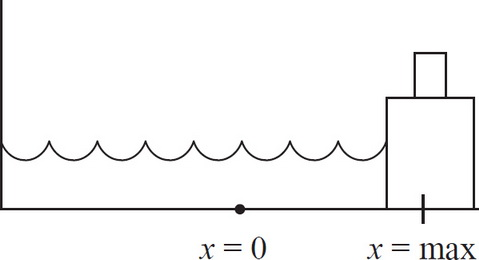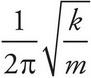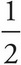# AP Physics 1 Question 287: Answer and Explanation

### Test Information

Question: 2875. A spring-block system is oscillating without friction on a horizontal surface. If a second block of equal mass were placed on top of the original block at a time when the spring is at maximum compression, which of the following quantities would NOT be affected? Assume that the top block stays on the bottom block.

• A. Frequency
• B. Maximum speed
• C. Amplitude
• D. All of the above quantities would be affected.

The frequency of a spring-block system is f =, so it would be affected by the change in mass. Furthermore, when a spring is at maximum compression or extension, all of its energy is potential energy, which is given by U =kx2. In adding this block, none of the relevant values are changed, so the spring will still extend to the same length, which means the amplitude is unchanged. Finally, maximum speed will be limited by the maximum K of the system (which will be unchanged since the maximum U was unchanged). K =mv2, so the increased mass would have to be balanced by a decrease in speed to leave the K unaltered.